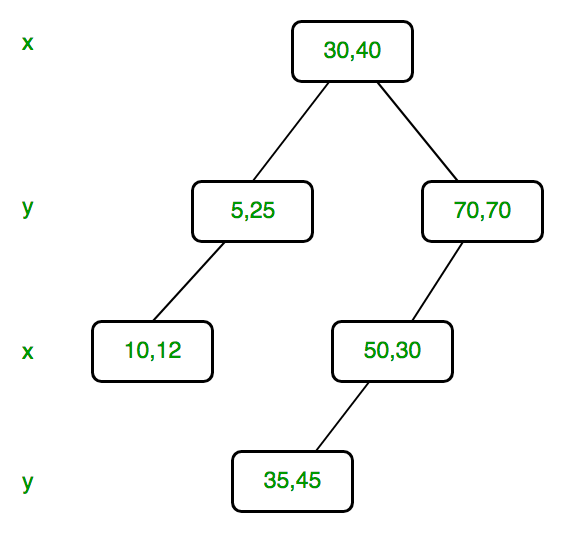Related Articles

# K Dimensional Tree | Set 2 (Find Minimum)

• Difficulty Level : Medium
• Last Updated : 03 Nov, 2020

We strongly recommend to refer below post as a prerequisite of this.

K Dimensional Tree | Set 1 (Search and Insert)

In this post find minimum is discussed. The operation is to find minimum in the given dimension. This is especially needed in delete operation.

For example, consider below KD Tree, if given dimension is x, then output should be 5 and if given dimensions is y, then output should be 12.In KD tree, points are divided dimension by dimension. For example, root divides keys by dimension 0, level next to root divides by dimension 1, next level by dimension 2 if k is more then 2 (else by dimension 0), and so on.

To find minimum we traverse nodes starting from root. If dimension of current level is same as given dimension, then required minimum lies on left side if there is left child. This is same as Binary Search Tree Minimum.
Above is simple, what to do when current level’s dimension is different. When dimension of current level is different, minimum may be either in left subtree or right subtree or current node may also be minimum. So we take minimum of three and return. This is different from Binary Search tree.

Below is C++ implementation of find minimum operation.

 `// A C++ program to demonstrate find minimum on KD tree``#include ``using` `namespace` `std;`` ` `const` `int` `k = 2;`` ` `// A structure to represent node of kd tree``struct` `Node {``    ``int` `point[k]; ``// To store k dimensional point``    ``Node *left, *right;``};`` ` `// A method to create a node of K D tree``struct` `Node* newNode(``int` `arr[])``{``    ``struct` `Node* temp = ``new` `Node;`` ` `    ``for` `(``int` `i = 0; i < k; i++)``        ``temp->point[i] = arr[i];`` ` `    ``temp->left = temp->right = NULL;``    ``return` `temp;``}`` ` `// Inserts a new node and returns root of modified tree``// The parameter depth is used to decide axis of comparison``Node* insertRec(Node* root, ``int` `point[], unsigned depth)``{``    ``// Tree is empty?``    ``if` `(root == NULL)``        ``return` `newNode(point);`` ` `    ``// Calculate current dimension (cd) of comparison``    ``unsigned cd = depth % k;`` ` `    ``// Compare the new point with root on current dimension 'cd'``    ``// and decide the left or right subtree``    ``if` `(point[cd] < (root->point[cd]))``        ``root->left = insertRec(root->left, point, depth + 1);``    ``else``        ``root->right = insertRec(root->right, point, depth + 1);`` ` `    ``return` `root;``}`` ` `// Function to insert a new point with given point in``// KD Tree and return new root. It mainly uses above recursive``// function "insertRec()"``Node* insert(Node* root, ``int` `point[])``{``    ``return` `insertRec(root, point, 0);``}`` ` `// A utility function to find minimum of three integers``int` `min(``int` `x, ``int` `y, ``int` `z)``{``    ``return` `min(x, min(y, z));``}`` ` `// Recursively finds minimum of d'th dimension in KD tree``// The parameter depth is used to determine current axis.``int` `findMinRec(Node* root, ``int` `d, unsigned depth)``{``    ``// Base cases``    ``if` `(root == NULL)``        ``return` `INT_MAX;`` ` `    ``// Current dimension is computed using current depth and total``    ``// dimensions (k)``    ``unsigned cd = depth % k;`` ` `    ``// Compare point with root with respect to cd (Current dimension)``    ``if` `(cd == d) {``        ``if` `(root->left == NULL)``            ``return` `root->point[d];``        ``return` `min(root->point[d], findMinRec(root->left, d, depth + 1));``    ``}`` ` `    ``// If current dimension is different then minimum can be anywhere``    ``// in this subtree``    ``return` `min(root->point[d],``               ``findMinRec(root->left, d, depth + 1),``               ``findMinRec(root->right, d, depth + 1));``}`` ` `// A wrapper over findMinRec(). Returns minimum of d'th dimension``int` `findMin(Node* root, ``int` `d)``{``    ``// Pass current level or depth as 0``    ``return` `findMinRec(root, d, 0);``}`` ` `// Driver program to test above functions``int` `main()``{``    ``struct` `Node* root = NULL;``    ``int` `points[][k] = { { 30, 40 }, { 5, 25 },``       ``{ 70, 70 }, { 10, 12 }, { 50, 30 }, { 35, 45 } };`` ` `    ``int` `n = ``sizeof``(points) / ``sizeof``(points);`` ` `    ``for` `(``int` `i = 0; i < n; i++)``        ``root = insert(root, points[i]);`` ` `    ``cout << ``"Minimum of 0'th dimension is "` `<< findMin(root, 0) << endl;``    ``cout << ``"Minimum of 1'th dimension is "` `<< findMin(root, 1) << endl;`` ` `    ``return` `0;``}`

Output:

```Minimum of 0'th dimension is 5
Minimum of 1'th dimension is 12```